1. 马尔科夫不等式

$P(X \ge t) \le \frac{E(X)}{t}$

2. 切比雪夫不等式

$Y = [X-E(X)]^2$

$P(Y \ge t^2) \le \frac{E(Y)}{t^2} = \frac{D(X)}{t^2}$

1. 马尔科夫（Markov）不等式
2. 切比雪夫（Chebyshev）不等式
3. 切尔诺夫（Chernoff）界

• 切尔诺夫界：『独立随机变量的和』的分布的尾概率的一般的界，通过矩生成函数得到，有『切尔诺夫型』、『指数形式』尾概率界等。
• 鞅：对不能抽象为『独立随机变量的和』的应用场景，鞅可以得到随机变量偏离期望的程度的界。

## 切尔诺夫界

### 矩生成函数

$M_X(t) = E\left[e^{t X}\right]$

\begin{aligned} E\left[e^{t X}\right] &= E\left[ \sum_{k=0}^{\infty} \frac{(tX)^k}{k!} \right] \\ &= \sum_{k=0}^{\infty}\frac{t^k}{k!}E\left(X^k\right) \end{aligned} \tag{1}

$(1)$ 式对 $t$$n$ 阶导，并令 $t = 0$，可以得到 $X$ 的各阶矩。

\begin{aligned} M_X^{(n)}(t) &= E(X^n) + \frac{1}{1}tE(X^{n+1}) + \frac{1}{2}t^2E(X^{n+2}) + \cdots \\ M_X^{(n)}(0) &= E(X^n) \end{aligned}

（另外一种更简单的证明在《概率与计算》上，直接利用期望和微分运算可以交换的性质。）

1. 矩生成函数相同，则随机变量分布相同。令 $X$$Y$ 是两个随机变量，如果对某个 $\delta > 0$，对于所有的 $t \in (-\delta, \delta)$$M_X(t) = M_Y(t)$，那么 $X$$Y$ 有相同的分布。

2. 随机变量的各阶矩相同，则这两个随机变量分布相同。

3. 独立随机变量的和的矩生成函数等于各自生成函数的积。

$E[e^{t(X+Y)}] = E[e^{tX} \cdot e^{tX}] = E[e^{tX}] \cdot E[e^{tY}]$

#### 两点分布和二项分布的矩生函数

\begin{aligned} M_X(t) &= E(e^{tX}) \\ &= p \cdot e^{t\cdot 1} + (1-p) \cdot e^{t\cdot 0} \\ &= 1-p+pe^t \end{aligned} \tag{2}

$E(X) = M_X^{(1)}(0) = \left(pe^t\right)|_{t=0} = p$

$E(X^2) = M_X^{(2)}(0) = \left(pe^t\right)|_{t=0} = p$

$D(X) = E(X^2) - E^2(X) = p - p^2 = p(1-p)$

\begin{aligned} M_Y(t) &= E(e^{tY}) \\ &= E\left(e^{t\sum_i^{n}{X_i}}\right) \\ &= E\left({e^{tX_i}}^n\right) \\ &= E\left({e^{tX_i}}\right)^n \\ &= [M_X(t)]^n \\ &= (1-p+pe^t)^n \end{aligned} \tag{3}

$M_Y^{(1)}(t) = n(1-p+pe^t)^{n-1} \cdot pe^t = npe^t(1-p+pe^t)^{n-1}$

\begin{aligned} M_Y^{(2)}(t) &= npe^t(1-p+pe^t)^{n-1} + npe^t \cdot (n-1)(1-p+pe^t)^{n-2} \cdot pe^t \\ &= npe^t(1-p+pe^t)^{n-1} + n(n-1)p^2e^{2t} \cdot (1-p+pe^t)^{n-2} \\ \end{aligned}

$E(Y) = [npe^t(1-p+pe^t)^{n-1}]|_{t=0} = np(1-p+p) = np$

\begin{aligned} E(Y^2) &= [npe^t(1-p+pe^t)^{n-1} + n(n-1)p^2e^{2t} \cdot (1-p+pe^t)^{n-2}]_{t=0} \\ &= np + n(n-1)p^2 \end{aligned}

\begin{aligned} D(Y) &= E(Y^2) - E^2(Y) \\ &= [np + n(n-1)p^2] - (np)^2\\ &= np + n^2p^2 - np^2 - n^2p^2 \\ &= np(1-p) \end{aligned}

### 切尔诺夫界的导出

$P[X \ge (1+\delta)\mu] < \left[\frac{e^\delta}{(1+\delta)^{1+\delta}}\right]^{\mu}$

$\mu=50$ 时，右式图像大概如此（使用 https://www.desmos.com/calculator 绘制，只看 $\delta>0$ 部分）：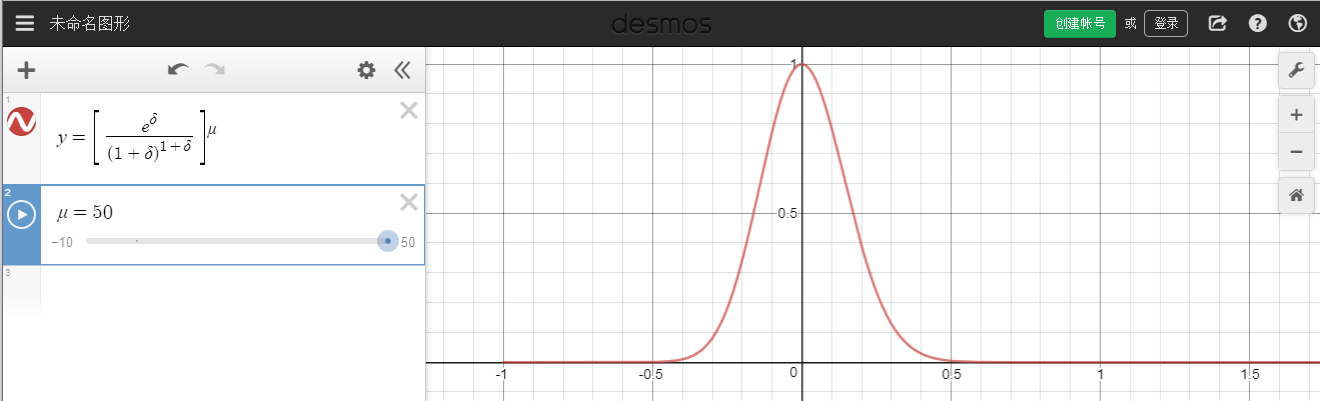1. 计算 $X$ 的矩生成函数

\begin{aligned} M_X(t) &\xlongequal{独立性} \prod_{i=1}^{n} M_{X_i}(t) \\ \end{aligned} \tag{4}

其中

\begin{aligned} M_{X_i}(t) &= P(X_i=1)e^{t\cdot 1} + P(X_i=0)e^{t\cdot 0} \\ &= p_ie^t + (1-p_i)e^0 \\ &= 1-p_i + p_ie^t \\ &\le e^{p_i(e^t-1)}, 这里用到了 1 + x \le e^x 在 R 上恒成立的条件 \end{aligned}

将放缩应用于 $(4)$ 式，得到一个比较好的形式：

\begin{aligned} M_X(t) &\xlongequal{独立性} \prod_{i=1}^{n} M_{X_i}(t) \\ & \le \prod_{i=1}^{n} e^{p_i(e^t-1)} \\ &= \exp\{(e^t-1)\sum_{i=1}^np_i\} \\ &= \exp \{(e^t-1)\mu\}, 这里用到了 \mu = E(X) = p_1 + \cdots + p_n \end{aligned}

2. 要计算 $P(X \ge t)$，等价于计算 $P(h(X) \ge h(t))$（在 $h$ 是增函数时）。应用 Markov 不等式 $P[h(X) \ge h(t)] \le \frac{E[h(X)]}{h(t)}$

\begin{aligned} P(X > t) &= P(e^{\lambda X} > e^{\lambda t}),注意 \lambda >0 满足增函数 \\ &\le \frac{E(e^{\lambda X})}{e^{\lambda t}} , 对随机变量 e^{\lambda X} 应用 Markov 不等式 \\ &\le \frac{\exp \{(e^\lambda-1)\mu\}}{\exp {\lambda t}} \end{aligned}

这里要尽量求出小的上界，因此取一个极小值：

\begin{aligned} P(X > t) &\le \min_{\lambda > 0} \frac{\exp \{(e^\lambda-1)\mu\}}{\exp {\lambda t}} \end{aligned}

3. 针对 $t$ 进行优化，得出结论

$t = (1+\delta)\mu$（为什么，因为概率内部取的是大于号 $X >t$，所以求偏离均值以上更有用，这样后面的 $\lambda$ 取特殊值才能保证是大于 0 的），有

\begin{aligned} P[X > (1+\delta)\mu] &\le \min_{\lambda > 0} \frac{\exp \{(e^\lambda-1)\mu\}}{\exp \{\lambda (1+\delta)\mu\}} \\ &令 \lambda = \ln(\delta+1)，既然小于所有中最小的，肯定也小于特例, \delta >0 \\ & \le \frac{e^{\delta\mu}}{(1+\delta)^{(1+\delta)\mu}} \\ &= \left[\frac{e^{\delta}}{(1+\delta)^{(1+\delta)}}\right]^\mu \\ \end{aligned} \tag{5}

$P[X \le (1-\delta)\mu] \le \left[\frac{e^{\delta}}{(1-\delta)^{(1-\delta)}}\right]^\mu \\ \tag{6}$

$(5)$ 和式 $(6)$ 总结起来就是：

$\begin{cases} P[X > (1+\delta)\mu] \le \left[\frac{e^{\delta}}{(1+\delta)^{(1+\delta)}}\right]^\mu, \delta > 0 \\ P[X \le (1-\delta)\mu] \le \left[\frac{e^{\delta}}{(1-\delta)^{(1-\delta)}}\right]^\mu, \delta \in (0, 1) \tag{7} \end{cases}$

### 切尔诺夫界的常用形式

$(7)$ 虽然精确，但是计算较复杂，我们可以得出一个可以忍受的、不太精确但是计算量较小的尾不等式：

$\begin{cases} P[X > (1+\delta)\mu] \le \left[\frac{e^{\delta}}{(1+\delta)^{(1+\delta)}}\right]^\mu \le \exp {\frac{-\mu\delta^2}{3}}, \delta \in (0, 1)（注意这里条件更强，限制 \delta 必须小于 1，下同） \\ P[X \le (1-\delta)\mu] \le \left[\frac{e^{\delta}}{(1-\delta)^{(1-\delta)}}\right]^\mu \le \exp {\frac{-\mu\delta^2}{2}}, \delta \in (0, 1) \tag{8} \end{cases}$

$\mu \ln \left[\frac{e^{\delta}}{(1+\delta)^{(1+\delta)}}\right] \le {\frac{-\mu\delta^2}{3}}$

$f(\delta) = (左边 - 右边)/\mu$，要证明 $f(\delta) \le 0$，由于 $f(0) = 0$，只需要证明 $f'(\delta) < 0$。求导即可。

\begin{aligned} f(\delta) &= \delta - (1+\delta)\ln (1+\delta) + {\frac{\delta^2}{3}} \\ &= \frac{\delta^2}{3} + \delta - (1+\delta)\ln(1+\delta) \\ \end{aligned}

\begin{aligned} f'(\delta) &= \frac{2}{3}\delta + 1 - \ln (1+\delta) - (1+\delta)\frac{1}{1+\delta} \\ &= \frac{2}{3}\delta-\ln (1+\delta) \end{aligned}

$f''(\delta) = \frac{2}{3} - \frac{1}{1+\delta}$

$f''(\delta)$ 是增函数，由于规定 $\delta \in (0, 1)$，所以 $f'' \in (-\frac{1}{3}, \frac{1}{6})$，因此 $f'$ 先减后增，要证明 $f' < 0$，只需求证两个端点 $f(0)$$f(1)$ 即可。

\begin{aligned} P[|X-\mu|\ge \delta\mu] & \le P[X \le (1-\delta)\mu] + P[|X \ge (1+\delta)\mu] \\ &= \exp {\frac{-\mu\delta^2}{2}} + \exp {\frac{-\mu\delta^2}{3}} \\ &= a +b \\ &\le 2b \\ &= 2\exp {\frac{-\mu\delta^2}{3}} \\ \tag{9} \end{aligned}

$P(X > t) < 2^{-t} \tag{10}$

\begin{aligned} P[X \ge (1+\delta)\mu] &\le \left(\frac{e^\delta}{(1+\delta)^{1+\delta}}\right)^\mu \\ &\le \left(\frac{e^{\delta+1}}{(1+\delta)^{1+\delta}}\right)^\mu \\ &= \left(\frac{e}{1+\delta}\right)^{(1+\delta)\mu} \\ & \le 2^{-(1+\delta)\mu} \\ &= 2^{-t} \end{aligned}

### 切尔诺夫界的简单应用

#### 成功实验的总次数

\begin{aligned} P[|X-\mu|\ge\delta\mu] &\le 2 \exp \{\frac{-\mu\delta^2}{3}\} \\ P[|X-\frac{n}{2}|\ge\frac{1}{2}\mu] &\le 2 \exp \{-\frac{\mu}{12}\} = 2\exp \{ -\frac{n}{24} \} \\ \end{aligned}

#### 算法重复遍数

1. 得到的解一定是正确的，
2. 运行时间不确定，运行时间与运行一次能够得到解得的概率 $p$ 有关。

$E(X) = \frac{1}{p}$

$E(|X-\mu|\ge \delta \mu) < 2 \exp \left\{-\mu\delta^2/3\right\} = p_0, \mu = \frac{1}{p}$

$\delta_0 = \sqrt{-3p(\ln p_0- \ln 2)}$

#### 参数估计

\begin{aligned} P(p \notin [q-\delta, q+\delta]) = P(pq+\delta) \end{aligned}

$p (p+\delta)n \Leftrightarrow X > (1+\delta/p)pn$

$p>q+\delta \Leftrightarrow p > X/n + \delta \Leftrightarrow X < (1-\delta/p)pn$

\begin{aligned} P(p \notin [q-\delta, q+\delta]) &= P[|X-\mu|>(\delta/p)\mu] \\ &\le 2\exp\{-\frac{\mu(\delta/p)^2}{3}\} \\ &= 2\exp\{ -\frac{n\delta^2}{3p}\} \\ &\le 2\exp\{ -\frac{n\delta^2}{3}\} \\ \end{aligned}

1. 令其为 $1-\gamma$，解得 $\delta_0$，得到置信区间
2. 知道置信区间，直接计算出置信水平
3. 直到置信区间和置信水平，可以得到实验所需次数

#### 【作业】 $\pi$ 值估计精度与抽样次数 $n$ 之间的关系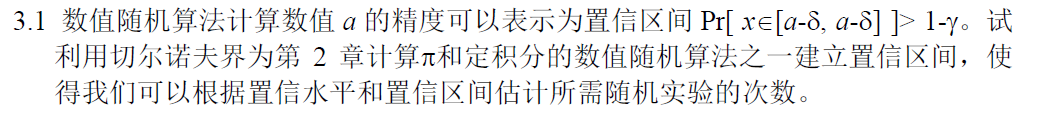\begin{aligned} P(p \notin [q-\delta, q+\delta]) = P(pq+\delta) \end{aligned}

$p (p+\delta)n \Leftrightarrow X > (1+\delta/p)pn$

$P(X > (1+\delta/p)pn) = P(X > (1+\delta/p)\mu) \le \exp \{-\frac{\mu(\delta/p)^2}{3}\} = \exp \{-\frac{pn(\delta/p)^2}{3}\} = \exp \{-\frac{n\delta^2}{3p}\}$

$p>q+\delta \Leftrightarrow p > X/n + \delta \Leftrightarrow X < (1-\delta/p)pn$

$P(X < (1-\delta/p)pn) = P(X < (1-\delta/p)\mu) \le \exp \{-\frac{\mu(\delta/p)^2}{2}\} = \exp \{-\frac{pn(\delta/p)^2}{2}\} = \exp \{-\frac{n\delta^2}{2p}\}$

\begin{aligned} P(p \notin [q-\delta, q+\delta]) &= P(pq+\delta) \\ &\le \exp \{-\frac{n\delta^2}{3p}\} + \exp \{-\frac{n\delta^2}{2p}\} \\ &= \exp \{-\frac{4n\delta^2}{3\pi}\} + \exp \{-\frac{4n\delta^2}{2\pi}\} \end{aligned}

$\exp \{-\frac{4n\delta^2}{3\pi}\} + \exp \{-\frac{4n\delta^2}{2\pi}\} = 1 - 0.95 = 0.05$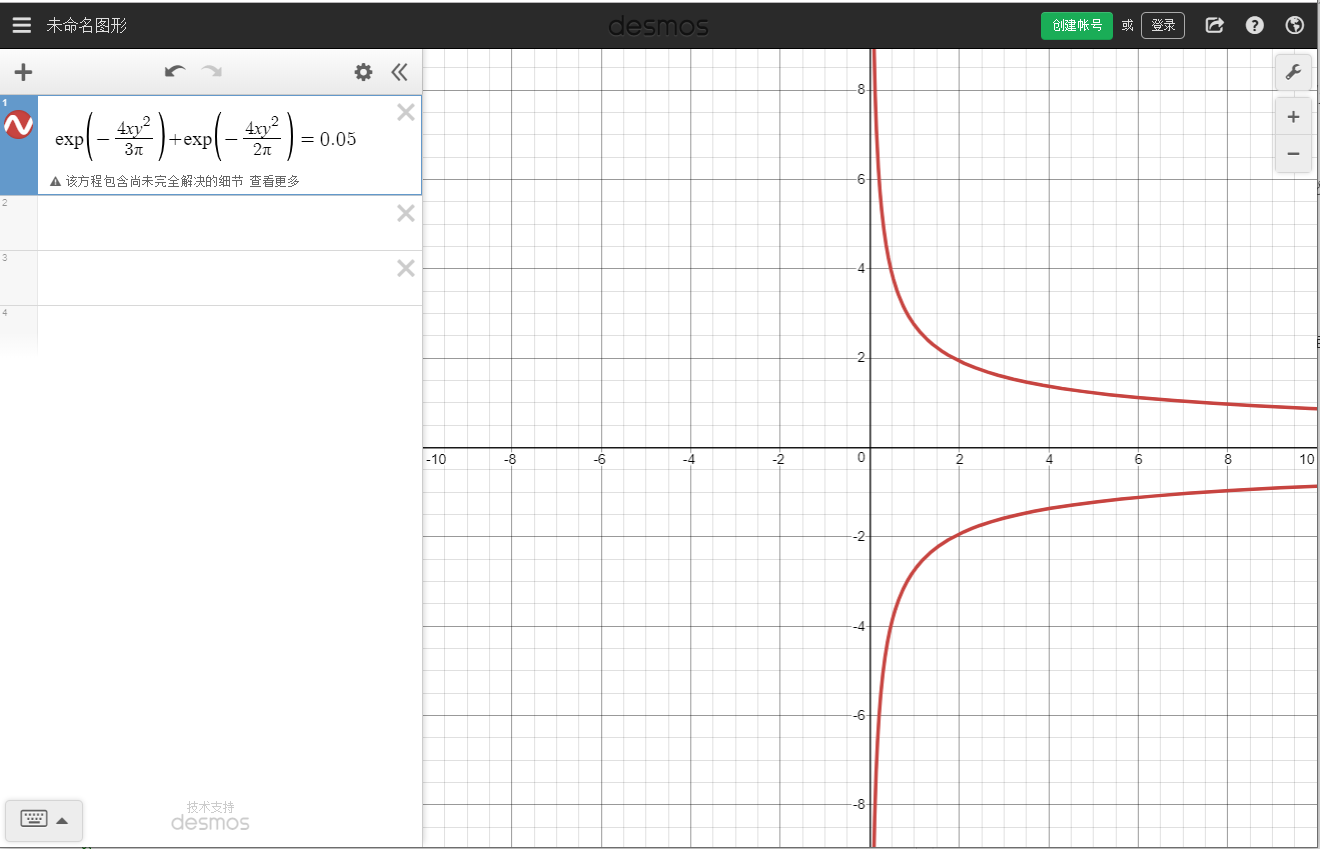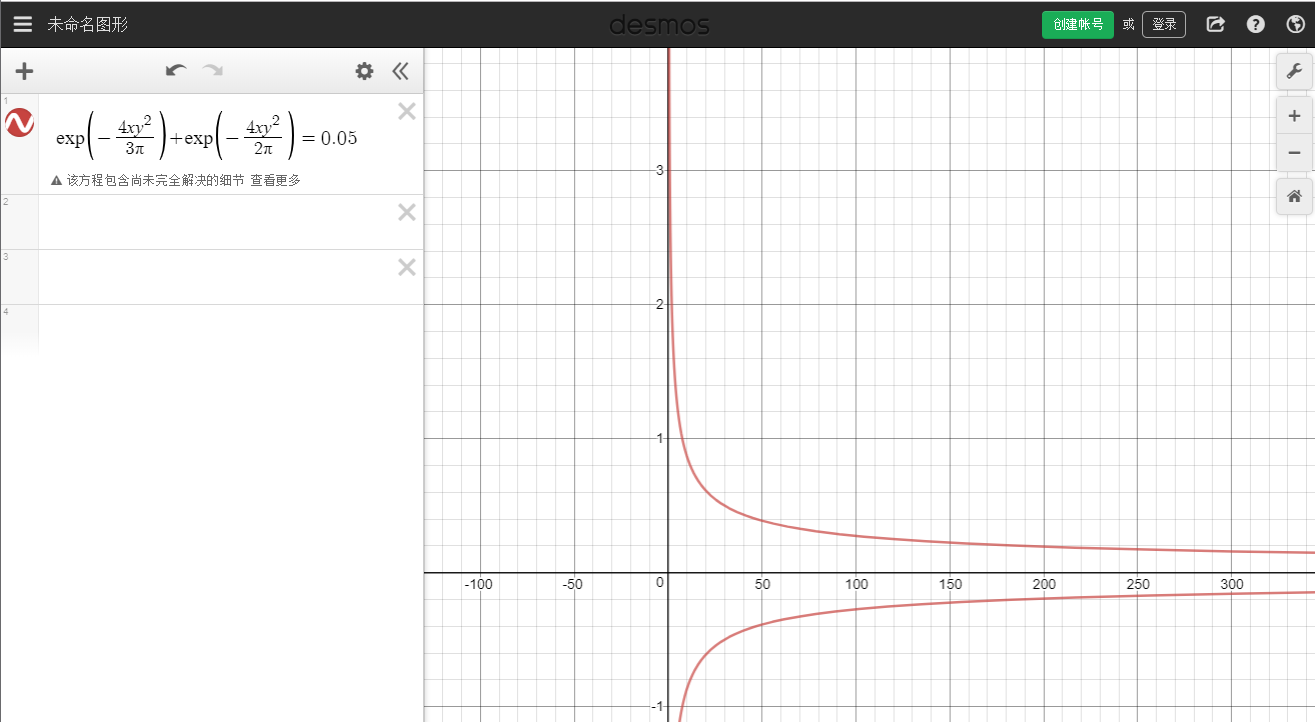#### 【作业】快排的随机性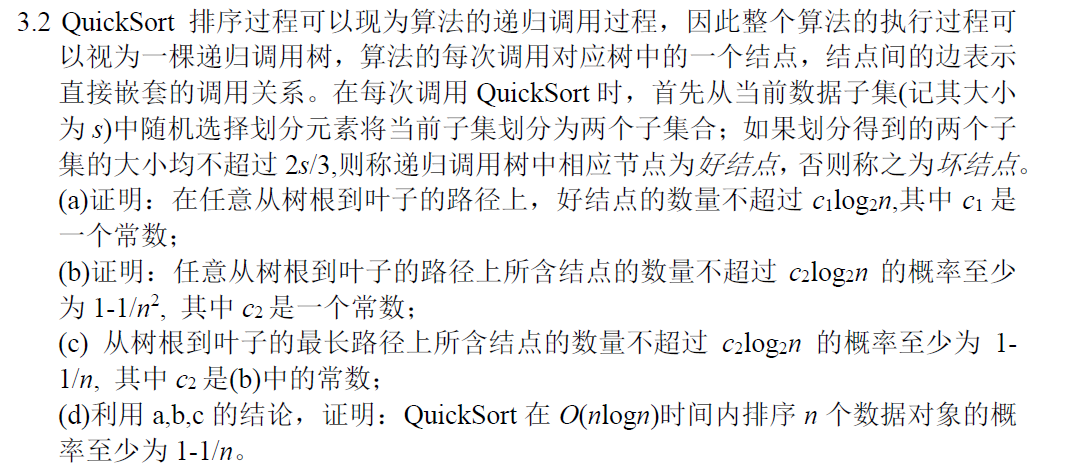(a)

$D(s)$ 表示 QuickSort 排序时所发生的动作对应的树的高度。我们用 $N(s)$ 表示排序 $s$ 个数对应的结点。对于树中有两个孩子 $N(a)$$N(s-a)$ 的结点 $N(s)$，我们有下面的递归等式：

$D(s) = \max \{D(a),D(s-a)\}+1$

（b)

$c_2 = 36$（也就是说，从树根到叶子的路径上所含结点的数量至少为 35）、$\delta = 9/20$。通过 $(a)$ 我们知道任意从根到叶子结点的路径上好结点的数量不超过 $c_1\log_2 n$，其中 $c_1 \approx 0.631$，可以推出坏结点的数量至少是 $35\log_2 n$。令 $X_i$ 表示一个指示随机变量，$X_i=1$ 表示第路径上第 $i$ 个结点是坏的，否则 $X_i=0$。我们要估计的概率就是 $P[X=\sum_{i=1}^{36\log_2 n}X_i \ge 35.369 \log_2 n]$。注意一个结点是好的当且仅当选中的基准元素大于等于第 $s/3$ 小的元素，或者小于等于第 $2s/3$ 小的元素。因此我们有 $P(X_i=1) \le 2/3, P(X_i=0)\ge 1/3$$E(X_i) \le (2/3) \cdot 36\log_2 n = 24 \log_2 n$。另外，根据切尔诺夫界，我们有：

$\mu_H = 24 \log_2 n$，我们有：

\begin{aligned} P[X \ge 35.369 \log_2 n] &\le P[X \ge 34.8 \log_2 n] \\ &= P[X \ge (1+\delta) \cdot \mu_H] \\ &\le \exp \{-\mu_H\delta^2/3\} \\ &= \exp \{-\frac{81}{50}\cdot\frac{\ln n}{\ln 2}\} \\ &\le n^{-2.337} \\ & < n^{-2} \end{aligned}

(d)

$T(n)$ 表示 Quicksort 排序 $n$ 个数时用的时间，我们有：

$T(n) = T(a) + T(n-a) + O(n)$

## 鞅

### 鞅的应用

$E(X_i|X_0,X_1,\cdots,X_{i-1}) = X_{i-1}, \forall i \ge 1$

#### 【习题】按照定义证明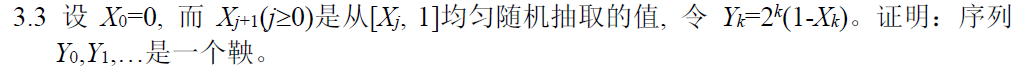$E(Y_i|Y_0,Y_1,\cdots,Y_{i-1}) = Y_{i-1}, \forall i \ge 1$

\begin{aligned} E(Y_i|Y_0,Y_1,\cdots,Y_{i-1}) &= E(2^i(1-X_i)|Y_0,Y_1,\cdots,Y_{i-1}) \\ &= 2^i - 2^iE[X_i|Y_0,Y_1,\cdots,Y_{i-1}] \end{aligned}

\begin{aligned} E(X_i|X_{i-1}) &= (X_{i-1}+1)/2 \end{aligned}

\begin{aligned} E(Y_i|Y_0,Y_1,\cdots,Y_{i-1}) &= 2^i - 2^iE[X_i|X_0,X_1,\cdots,X_{i-1}] \\ &= 2^i - 2^iE[X_i|X_{i-1}] \\ &= 2^i - 2^i\frac{X_{i-1}+1}{2} \\ &\xlongequal{带回去} 2^i\left(1-\frac{1-\frac{Y_{i-1}}{2^{i-1}}+1}{2}\right) \\ &= Y_{i-1} \end{aligned}

#### 【习题】应用-分析时间复杂度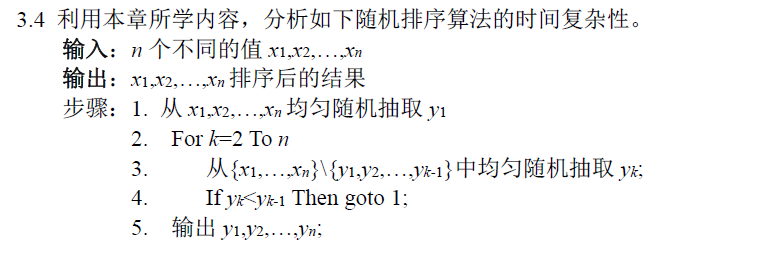• 成功的概率为 $p$，所用的时间为 $O(n)$
• 不成功的概率为 $1-p$，所用的时间为 $O(J_i)$$i$ 表示第几次执行，其中 $J_i$ 为第一个满足 $y_k < y_{k-1}$$k$$J$ 的期望为 $E(J_i)$

$E(X) = \frac{1}{p}$

$T = \sum_{i=1}^{X-1}O(J_i) + O(n)$

$E\left(\sum_{i=0}^N X_i\right) = \sum_n P(N=n) \, E\left(\sum_{i=0}^n X_i\right)$

\begin{aligned} E(T) &= E\left[\sum_{i=1}^{X-1}O(J_i) + O(n)\right] \\ &= E\left[\sum_{i=1}^{X-1}O(J_i)\right] + O(n) \\ &= \sum_{x} P(X=x)E\left(\sum_{i=1}^{x-1}O(J_i) \right) + O(n) \\ & 每一轮运行 J 的均值都是一样的，记为 E(J) \\ &= \sum_{x} P(X=x)(x-1)O\left[E(J) \right] + O(n) \\ &= O\left[E(J) \right]\sum_{x} P(X=x)(x-1) + O(n) \\ &= O\left[E(J) \right][E(X)-1] + O(n) \\ &= O[E(J)](\frac{1}{p}-1) + O(n) \\ \end{aligned}

1. 所有可能的排序中只有 1 种是正确的（不考虑元素相等的情况），成功的概率 $p = \frac{1}{n!}$
2. 『第 $i$ 元素才开始打破有序性，前 $(i-1)$ 个元素均有序』的概率为 $P(J = i)$，前 $(i-2)$ 个元素只有 1 种排法，第 $(i-1)$ 个元素有两种排法，其中一种排法不管第 $i$ 个元素选择什么都会打破有序性，另外一种排法要打破有序性必须第 $i$ 个元素只有 $(n-i)$ 种排法，第 $(i+1)$ 个及后面的元素排法任意，$P(J = i) = \frac{[(n-i+1)! + (n-i)(n-i)!]}{n!} < \frac{2(n-i+1)!}{(n)!}$。因此 $E(J) = \sum_{j=2}^{n}P(J=j) j < \sum_{j=2}^{n}\frac{2(n-j+1)!}{n!}j \le \sum_{j=2}^{n}\frac{2(n-2+1)!}{n!}j \le \sum_{j=2}^{n}j = \frac{(n-1)(n+2)}{2}$

\begin{aligned} E(T) &= O\left( \sum_{j=2}^n\frac{j}{C_n^j}(n!-1) + n \right) \\ &\le O\left[ O(n)(n!-1) + n \right] \\ &= O[(n+1)!] \end{aligned}

$T = \sum_{i=1}^{X-1}O(J_i) + O(n)$

$E[\sum_{i=1}^{X-1}O(J_i)] = E(X-1)\cdot E(J)$